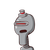# D. Determine if the events are mutually exclusive or non-mutually exclusive.Then, solve the problem completely.1. Find

D. Determine if the events are mutually exclusive or non-mutually exclusive.
Then, solve the problem completely.
1. Find the probability of selecting a boy cr a blond-haired person from 12
girls, 5 of whom have blond hair, and 15 boys, 6 of whom have blond hair.
2. Find the probability of drawing a king or queen from a standard deck of
cards.
3. Find the probability of two dice being tossed and showing a sum of 6 or a
sum of 9
4. A weather forecaster states that the probability of rain is 3/5, the
probability of lightning is 2/5, and the probability of both is 1/5. What is
the probability of a sporting event being cancelled due to rain or lightning?
5. A bag contains cards numbered from 1 to 14. One card is drawn at random.
Find the probability of selecting a prime number or a multiple of four.​

### 1 thought on “D. Determine if the events are mutually exclusive or non-mutually exclusive.<br />Then, solve the problem completely.<br />1. Find”

1.